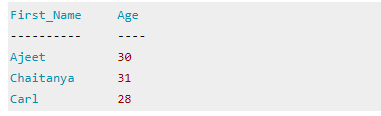FREE E LEARNING PLATFORM

# DBMS Relational Calculus

Relational calculus is a non-procedural query language that tells the system what data to be retrieved but doesn’t tell how to retrieve it.

## Types of Relational Calculus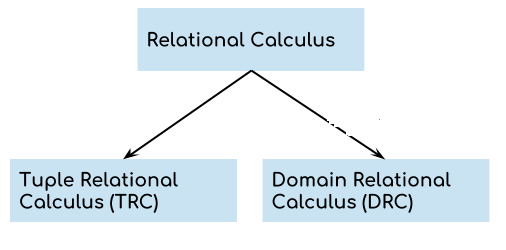## 1. Tuple Relational Calculus (TRC)

Tuple relational calculus is used for selecting those tuples that satisfy the given condition.

Table: Student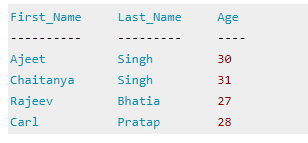Lets write relational calculus queries.
Query to display the last name of those students where age is greater than 30
{ t.Last_Name | Student(t) AND t.age > 30 }

In the above query you can see two parts separated by | symbol. The second part is where we define the condition and in the first part we specify the fields which we want to display for the selected tuples.

The result of the above query would be: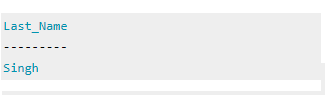Query to display all the details of students where Last name is ‘Singh’

{ t | Student(t) AND t.Last_Name = 'Singh' }

## Output: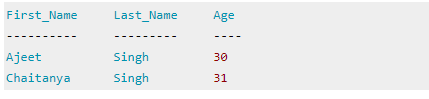## 2. Domain Relational Calculus (DRC)

In domain relational calculus the records are filtered based on the domains. Again we take the same table to understand how DRC works.

Table: Student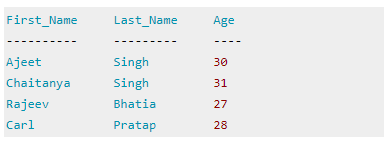Query to find the first name and age of students where student age is greater than 27

{< First_Name, Age > | ∈ Student ∧ Age > 27}

Note: The symbols used for logical operators are: ∧ for AND, ∨ for OR and ┓ for NOT.

## Output: